Dr. J's Maths.com
Where the techniques of Maths
are explained in simple terms.

Applications of Calculus - Equations of motion in one direction.
Test Yourself 2.

 The questions on this page focus on: Given a displacement equation; Equation uses a log function; Equation uses an exponential function; Equation uses a trig function; Given a velocity equation; Given an acceleration equation; Movement of 2 particles.

 Given a displacement equation 1. The displacement of a particle moving along the x-axis is described by the equation x = t4 + 4t3 - 20t2 + 1 where x is the displacement from the origin in metres and t is the time in seconds. (i) Describe the initial motion of the particle. (ii) Describe how the motion of the particle has changed by t = 2 seconds. (iii) At what time(s) is the particle stationary but accelerating towards the right? Answers(i) At t = 0 sec, particle is at the origin with zero velocity and acceleration of 1 m/sec2. (ii) At t = 1 sec, particle is 14 metres to the left of the origin, velocity = -24 m/sec and with an acceleration of -4 m/sec2 (to the left). (iii) Particle has zero velocity but positive acceleration at t = 2. 2. 3. 4. Log function 5. A particle moves in a straight line such that its position at time t seconds is given by x = t - loget. (i) When is the particle at rest? (ii) Find the exact distance traveled by the particle between t = 0.5 secs and t = 1.5 secs. Answers(i) At rest at t = 1 sec. (ii) Distance = log(4/3) m. 6. The displacement x metres of a particle moving in a straight line at time t seconds is given by x = 2t - 4 loge(2t + 1). (i) Find the initial velocity of the particle. (ii) Show that the acceleration of the particle is always positive. Answers(i) Initial velocity is -6 m/sec. 7. Exponential eqn. 8. The equation of motion of a particle is x = te-t where x is in centimetres and t is in seconds. (i) Find the time when the particle is at rest. (ii) Find the equation of motion for acceleration and the acceleration when v = 0. (iii) Find the time when the acceleration is zero. (iv) Sketch the displacement-time function showing all relevant information especially related to your answers above. Answer.(i) t - 1.(ii) Accel = (t - 2)e-t.(iii) Acceleration = 0 when t = 2. 9. The equation for the velocity of a particle moving in a straight line iswith t measured in minutes and v expressed as ms-1. The particle is initially at the origin. (i) What is the particle's initial velocity? (ii) Develop an equation for the displacement x of the particle. (iii) Show that when x = 8,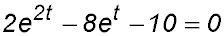. (iv) Hence find when the particle is at x = 8 metres. Answer.(iv) t = ln 5. 10. Trig function. 11. A particle is moving in a straight line. At time t seconds, its distancex from a fixed point P is goven by the equation: x = 2 + 2sin(πt/4) (i) Calculate the initial position of the particle. (ii) Find the exact velocity of the particle after 1 scond. (iii) Find the position of the particle when it is stationary for the second time. Answer.(i) x = 2.(ii) v = π/(2√2)(iii) At t = 6, x = 2. 12. A particle moves along a straight line so that its distance x metres from a fixed point O is given by x = t + cos t where t is the time in seconds. (i) Where was the particle initially? (ii) When and where does the particle first come to rest? (iii) When does the particle next some to rest? (iv) What is the acceleration of the particle after π/6 seconds? Answers(i) At x = 1. (ii) At x = π/2 when t = π/2.(iii) At t = 5π/2 secs. (iv) Accel = -√3/2 m/sec2 13. The motion of a particle moving in a straight line is described by the function x = 3cos 2t where x is the displacement (in metres) and t is the elapsed time (in seconds). (i) Draw a sketch of the displacement-time graph from t = 0 to t = π. (ii) Find an expression for the particle's velocity. (iii) In which direction is the particle moving after 2 seconds? (iv) The particle is initially at rest. Find when it next comes to rest. (v) Show that the acceleration of the particle can be described by a = -4x. (vi) Find the maximum displacement of the particle. (vii) By drawing an appropriate line on your graph, find the number of occasions in this time period when the particle will have a displacement of 2. Answer. (ii) v = -6sin 2t.(iii)v = 4.54 - so in a positive direction. (iv)Next at rest at π/2.(vi)Amplitude = 3 m.(vii) On 2 occasions. 14. A particle moves along a straight line so that its distance x metres from a fixed point O is given by x = sin 2t - t where t is measured in seconds. (i) What is the initial velocity of the particle? (ii) When and where does the particle first come to rest? (iii) What is the acceleration of the particle when t = π/8 seconds? (iv) What is the distance the particle has traveled when it is at rest for the second time? (iv) What is the displacement of the particle at t = 3π seconds? Given velocity equation 15. A particle moves in a straight line. Its velocity in metres/second after t seconds is given by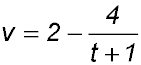. (i) Find the initial velocity. (ii) Find the acceleration of the particle when the particle is stationary. (ii) By considering the behaviour of v for large t, sketch a graph of v against t for t ≥ 0 showing any intercepts. (iv) Find the exact distance traveled by the particle in the first 7 seconds. Answer.(i) At t = 0, v = -2. (ii) Stationary at t = 1 so accel. = 1 m/sec2 (iv) Distance = 10 - 4ln 2. 16. The velocity, in metres/second, of a particle moving in a straight line, at t seconds is given by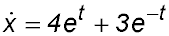. Initially the particle is at the origin. (i) What is the initial acceleration of the particle? (ii) Show that when the particle is 10 m to the right of the origin, then 4e2t - 11et - 3 = 0. (iii) Hence find the time when x = 10. 17. A particle is moving in a straight line with velocity v = 3et - 12e-2t Initially the particle is at the origin. Time t is measured in seconds and velocity v is measured in metres/second. (i) Find the initial velocity of the particle. (ii) Is the particle ever at rest? Explain your answer. (iii) Find the displacement of the particle at t = 4. Write your answer correct to the nearest metre. Answer.(i) initial velocity is -9 cm/sec. (ii) Yes at rest at (ln 4)/3 sec. (iii) Distance = 155 m. 18. A particle moves in a straight line so that after t seconds (t ≥ 0) its velocity v is given byThe displacement of the particle from the origin is v=given by x metres. (i) Find the acceleration of the particle when t = 0. (ii) If the particle is initially at the origin, find the displacement as a function of t. (iii) When is the particle stationary? (iv) How far does the particle travel in the first 2 seconds? 19. A particle is moving in a straight line. At time t seconds, its displacement is x metres from a fixed point O. Its velocity is described by the equation v = 3t2 - 2t -1. Initially the particle is 1 metre to the right of O. (i) Show that the particle is at rest after 1 second. (ii) Find the displacement x in terms of t. (iii) Find the distance travelled by the particle in the first 2 seconds of its motion. 20. A particle, initially 12/π metres to the left of the origin moves along a straight line about a fixed point O so that its velocity v m/sec at time t seconds is given by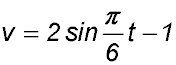(i) Find the velocity of the particle when t = 6 seconds. (ii) Show that the particle is first at rest when t = 1 second. (iii) Find the acceleration of the particle when it is first at rest. (iv) Find the greatest speed of the particle. 21. The velocity of a particle moving in a straight line is given by the equation v = (t - 2)2 + 5 m/sec. (i) Find the minimum speed of the particle. (ii) Find the initial acceleration of the particle. (iii) Find the distance travelled by the particle in the first 3 seconds. (iv) Initially the particle is 9 m to the left of the origin. Find the position of the particle at t = 5. Given acceleration equation. 22. A particle is moving with acceleration described by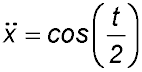. It is initially at the origin and moving with a velocity of 2 m/sec (i) By determining its velocity equation, show that the particle was stationary for the first time at t = 3π seconds. (ii) How far did the particle move before it became stationary? Answer.(ii) 6π + 4 = 22.85 secs. 23. The graph below shows the acceleration of a particle during a 6 second interval.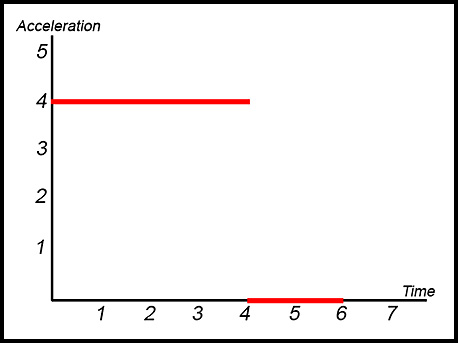Initially the particle is at rest at the origin. (i) Sketch the velocity-time graph of the particle for 0 ≤ t ≤ 6. (ii) Calculate the distance traveled by the particle during this 6 second interval. Answer.(ii) 64 m. Two particles